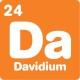# What's new## Binary for beginners

, 14 January 2014

Binary is a way of writing numbers using two digits, typically represented by 1 and 0. It takes some practice to be able to read binary, but it’s the basis of how almost all computers do arithmetic!

A binary number looks like a standard decimal number, but it only has the digits 1 and 0. To distinguish binary from standard numbers, we’ll put a ‘B’ on the end of any binary numbers. Some people will instead write “0b” at the start, or “2” at the end to show the same thing.

A standard number has a ones column, a tens column, a hundreds column and so on. If binary used the same values for each column, the only numbers would be 1, 10, 11, 100 and so on.

Binary columns are valued differently. A binary number has a ones column, a twos column, a fours column, an eights column, and so on. Each column is twice the previous column. To read a binary number, add the values of the columns together. For example:

1011B

Has 1 in the ones column, 1 in the twos column, 0 in the fours column and 1 in the eights column, so

1011B = one + two + eight = eleven

And for a bigger example:

1000110B = two + four + sixty-four = seventy

Binary addition is based on one simple fact:

1B + 1B = 10B

To do binary addition, write the numbers with their columns lined up like you would with regular numbers:

111B

+ 110B

Then add the columns, starting from the ones column and move up. The following three facts should help you:

0B + 0B = 0B
1B + 0B = 1B
1B + 1B = 10B

Don’t forget to carry when you add two 1s together!

111B

+ 110B

––––––

1101B

To check your answer, you can convert it to standard decimal numbers:

111B = seven, 110B = six, 1101B = thirteen, so:

Seven + six = thirteen

### What’s happening?

If you do this activity, you might think that binary is complicated and slow. In the example above, it takes four steps to add six and seven. However, most arithmetic in the world is done in binary – because most arithmetic is done by computers.

Binary numbers are written with only two digits: 1 and 0. A computer can store a binary number using open or closed switches to represent each digit. It is also easier to design chips to add binary. There are only three addition facts needed to make a binary adder work (0 + 0 = 0, 1 + 0 = 1, 1 + 1 = 10). A decimal adding machine would need 55 facts.

The binary system was formally described by Gottfried Leibniz about 300 years ago. Gottfried based his binary system on ideas he found in a fortune telling system known as I Ching. Leibniz though that binary would make a good system for computation, but it was hundreds of years before the first binary computers were made.

If you’re after more maths activities for kids, subscribe to Double Helix magazine!Categories:

## Similar posts

1.David, I love your content. Me and my two friends would like you to make an article about hexadecimal because you’re very helpful. I hope you have an amazing day!

2.1st former here cant understand this t all and project due tmrw

3.Hi Josiah,
4.